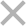自考导航

# 2020年10月上海自考经济类管理会计(一)复习资料五

【导语】1、单位贡献毛益=单价-单位变动制造成本

2、单位贡献毛益=单价-单位变动制造成本-单位变动销售及管理费用

(1)甲产品的销售比重=2000×60/200 000=60%，乙产品的销售比重=500×30÷200 000=7.5%，丙产品的销售比重=1000×65÷200 000=32.5%.

(2)营业利润=贡献毛益-固定成本=销售收入×贡献毛益率-固定成本=(2000×60+500×30+1000×65)×24.75%-19800=49500-19800=29700元

1、计算指标：

(1)盈亏临界点的销售额=72000/36%=200000元

(2)A产品在盈亏临界点的销售量=200000×0.6/5=24000件

B产品在盈亏临界点的销售量=200000×0.4/2.50=32000件

(3)安全边际额=5×30000+2.50×40000-200000=50000元

(4)预计利润=50000×36%=18000元

2、增加A产品销量引起贡献毛益增加=(40000-30000)×5×40%=20000元，减少B销售量引起的贡献毛益减少=(40000-32000)×2.5×30%=6000元，合计引起的贡献毛益的增加为20000-6000=14000元，与增加的广告费9700元(固定成本)相比，超出4300元，所以方案合算。

3、重新计算

A产品销售额=200000元，B销售额=80000元### 上海自考微信公众号